ISSN: 1070-4698

# Vol. 31

Latest Volume
All Volumes
All Issues

PIER Vol. 31, 311-329, 2001. doi:10.2528/PIER00071705

###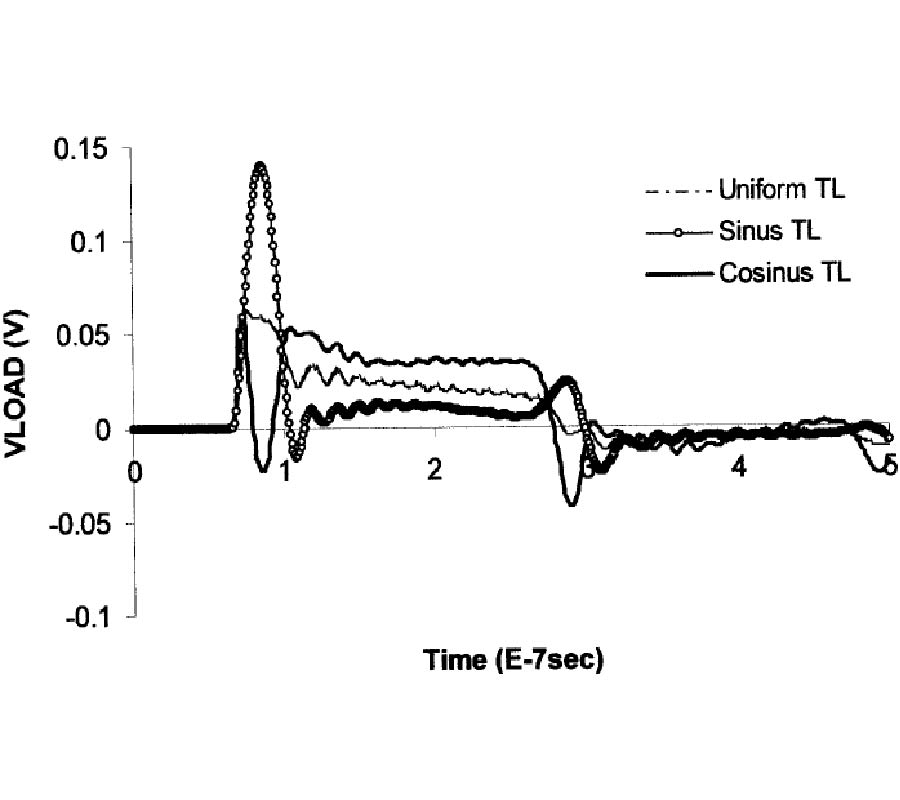Validation of a Modified FDTD Method on Non-Uniform Transmission Lines

P. Trakadas and Christos N. Capsalis

In this paper, we introduce a modified FDTD model in order to investigate the behavior of the induced voltage of a non-uniform transmission line (TL) excited by lumped voltage sources or external electromagnetic wave. The parameters to be taken into account for this specific coupling phenomenon are explicitly analyzed and the affection on the behavior of the induced voltage is discussed in detail. To confirm the validity of this model, results obtained by this model, for two typical transmission line configurations, are compared to results obtained by other models, already published in the literature. Finally, several numerical calculations of the line responses are provided for non-uniform TL's that can be present in practical configurations. These results indicate that a configuration slightly different from the uniform one can cause large discrepancies on the termination voltages of the TL.

PIER Vol. 31, 291-310, 2001. doi:10.2528/PIER00071702

###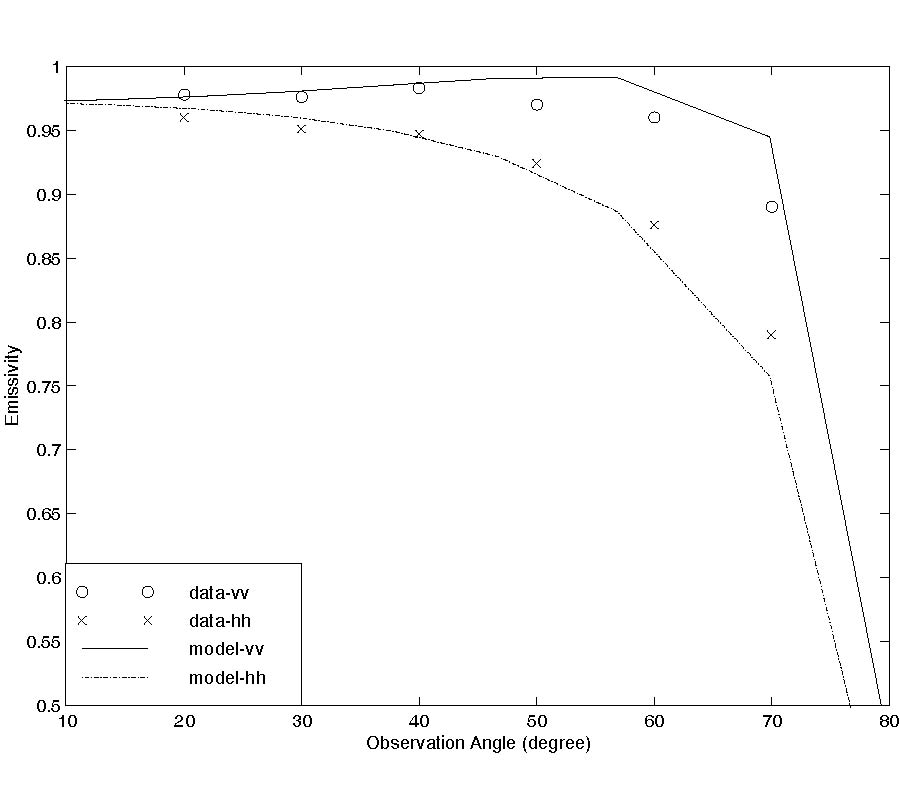Microwave Emission Model for Wet Snow by Using Radiative Transfer and Strong Fluctuation Theory

H. Wang, Ali Arslan, Jouni Pulliainen, and M. Hallikainen

This study is concerned with the development of a model to describe microwave emission from terrain covered by wet snow. The model is based on the radiative transfer theory and the strong fluctuation theory. Wet snow is treated in the model as a mixture of dry snow and water inclusions. The shape of the water inclusions is taken into account. The effective permittivity is calculated by using the two-phase strong fluctuation theory model with nonsymmetrical inclusions. The phase matrix and the extinction coefficient of wet snow for an anisotropic correlation function with azimuth symmetric are used. The vector radiative transfer equation for a layer of a random medium was solved by using Gaussian quadrature and eigen analysis. The model behaviour is illustrated by using typical parameters encountered in microwave remote sensing of wet snow. Comparisons with emissivity data at 11, 21 and 35 GHz are made. It is shown that the model predictions fit the experimental data.

PIER Vol. 31, 273-290, 2001. doi:10.2528/PIER00071709

###Effective Permittivity of Wet Snow Using Strong Fluctuation Theory

Ali Arslan, H. Wang, Jouni Pulliainen, and M. Hallikainen

The strong fluctuation theory is applied to calculate the effective permittivity of wet snow by a two-phase model with nonsymmetrical inclusions. In the two-phase model, wet snow is assumed to consist of dry snow (host) and liquid water (inclusions). Numerical results for the effective permittivity of wet snow are illustrated for random media with isotropic and anisotropic correlation functions. A three-phase strong fluctuation theory model with symmetrical inclusions is also presented for theoretical comparison. In the three-phase model, wet snow is assumed to consist of air (host), ice (inclusions) and water (inclusions) and the shape of the inclusions is spherical. The results are compared with the Debye-like semi-empirical model and a comparison with experimental data at 6, 18 and 37 GHz is also presented. The results indicate that (a) the shape and the size of inclusions are important, and (b) the two-phase model with non-symmetrical inclusions provides the good results to the effective permittivity of wet snow.

PIER Vol. 31, 247-272, 2001. doi:10.2528/PIER00081601

###Dual Frequency Polarimetric SAR Data Classification and Analysis

Laurent Ferro-Famil and Eric Pottier

In this paper, we introduce a new classification scheme for dual frequency polarimetric SAR data sets. A (6×6) polarimetric coherency matrix is defined to simultaneously take into account the full polarimetric information from both images. This matrix is composed of the two coherency matrices and their cross-correlation. A decomposition theorem is applied to both images to obtain 64 initial clusters based on their scattering characteristics. The data sets are then classified by an iterative algorithm based on a complex Wishart density function of the 6 by 6 matrix. A class number reduction technique is then applied on the 64 resulting clusters to improve the efficiency of the interpretation and representation of each class characteristics. An alternative technique is also proposed which introduces the polarimetric cross-correlation information to refine the results of classification to a small number of clusters using the conditional probability of the crosscorrelation matrix. The analysis of the resulting clusters is realized by determining the rigorous change in polarimetric properties from one image to the other. The polarimetric variations are parameterized by 8 real coefficients derived from the decomposition of a special unitary operator on the Gell-Mann basis. These classification and analysis schemes are applied to full polarimetric P, L, and C bands SAR images of the Nezer forest acquired by NASA/JPL AIRSAR sensor (1989).

PIER Vol. 31, 225-245, 2001. doi:10.2528/PIER00071706

###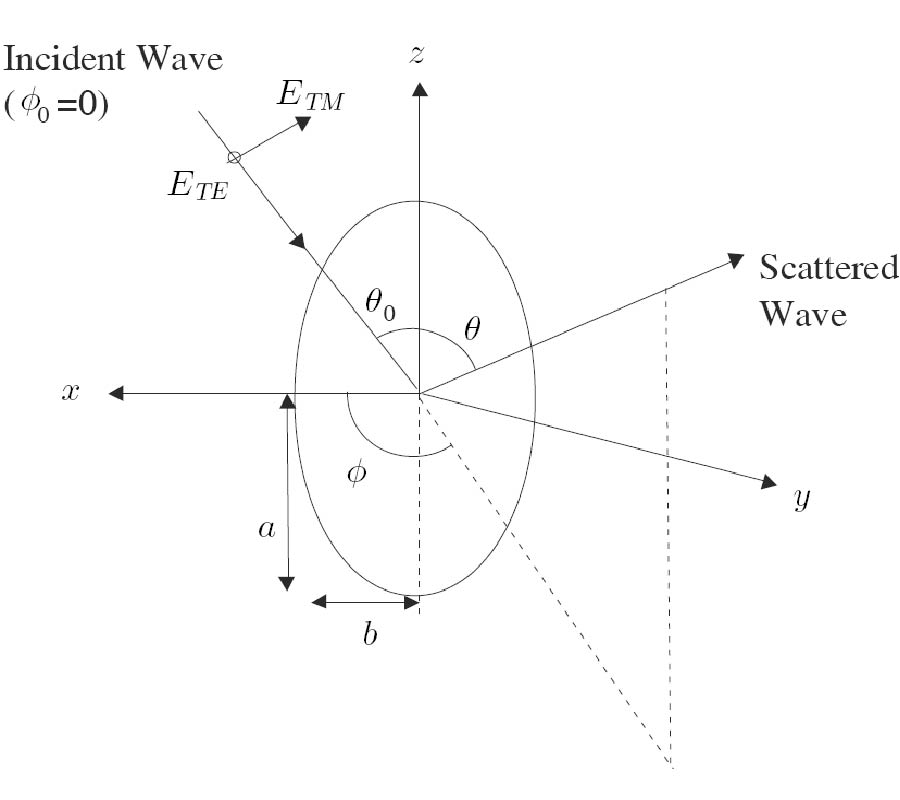Bistatic Scattering and Backscattering of Electromagnetic Waves by Conducting and Coated Dielectric Spheroids: a New Analysis Using Mathematica Package

L.-W. Li, T. S. Yeo, and M.-S. Leong

Solutions to electromagnetic scattering at any angle of incidence by a perfectly conducting spheroid and a homogeneous dielectric spheroid coated with a dielectric layer are obtained by solving Maxwell's equations together with boundary conditions. The method used is that of expanding electric and magnetic fields in the spheroidal coordinates in terms of the spheroidal vector wave functions and matching their respective boundary conditions at spheroidal interfaces. In this formulation, the column vector of the series expansion coefficients of the scattered field is obtained from that of the incident field by means of a matrix transformation, which is in turn obtained from a system of equations derived from boundary conditions. The matrix depends only on the scatterer's properties; hence the scattered field at a different direction of incidence is obtained without repeatedly solving a new set of simultaneous equations. Different from the previous work, the present work developed an accurate and efficient Mathematica source code for more accurate solution to the problem. Normalized bistatic and backscattering cross sections are obtained for conducting (for verification purpose), homogeneous dielectric (for verification purpose), and coated dielectric prolate (for some new results) spheroids, with real and complex permittivities. Numerically exact results for the coated dielectric prolate spheroids are newly obtained and are not found in existing literature.

PIER Vol. 31, 195-223, 2001. doi:10.2528/PIER00062901

### Computationally Efficient Expressions of the Dyadic Green's Function for Rectangular Enclosures

Filippo Marliani and A. Ciccolella

This paper considers expressions of the dyadic Green's function for rectangular enclosures, which are efficient from a computational point of view. The inherent application is to solve numerically electromagnetic scattering problems with an integral equation formulation, using the Method of Moments. The Green's dyadic is derived from an image-spectral approach, which has the flexibility to generate directly the expression with the fastest convergence once the locations of both the observation and the source points are given. When the observation point is at the source region, slowly converging sums arise that are overcome by extending the method of Ewald to the dyadic case. Numerical proofs are reported in tabular form to validate the technique developed.

PIER Vol. 31, 181-193, 2001. doi:10.2528/PIER00062202

###Near Fields of Electrically Small Thin Square and Rectangular Loop Antennas

L.-W. Li, C.-P. Lim, and Mook-Seng Leong

This paper presents an alternative vector analysis of the electromagnetic (EM) fields radiated from electrically small thin square and rectangular loop antennas of arbitrary length 2a and width 2b . This method employs the dyadic Green's function (DGF) in the derivation of the EM radiated fields and thus makes the analysis general, compact and straightforward. Both near- and far-zones are considered so that the EM radiated fields are expressed in terms of the vector wave eigenfunctions. Not only the exact solution of the EM fields in the near and far zones outside the region (where r > √a2 + b2 ) is derived by use of spherical Hankel functions of the first kind, but also the closed series form of the EM fields radiated in the near zone inside the region 0 ≤ r < b is obtained in series of spherical Bessel functions of the first kind. The two regions between the radial distances b and √a2 + b2 which are defined as intermediate zones are characterized by both the spherical Bessel and Hankel functions of the first kind. Validity of the numerical results is discussed and clarified.

PIER Vol. 31, 155-179, 2001. doi:10.2528/PIER00062201

###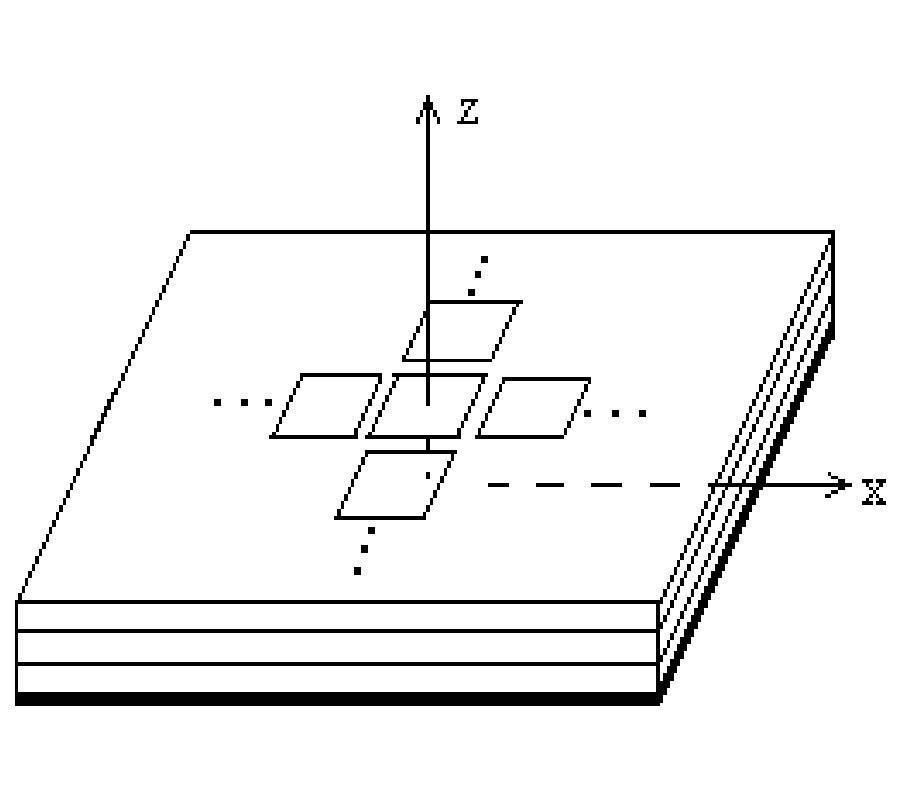A Method of Moments Analysis of a Microstrip Phased Array in Three-Layered Structures

Z.-F. Liu, P.-S. Kooi, L.-W. Li, M.-S. Leong, and T. S. Yeo

In this paper, the scanning characteristics of an infinite stacked microstrip phased array in a three-layered structure are analysed. In the analysis of the field distribution, the spectral-domain Galerkin Method of Moments together with the planar dyadic Green's function is applied. An attachment mode current is employed to model the singularity of currents nearby the feed point so as to facilitate the fast convergence. The currents on all patches are expanded in terms of the trigonometric basis and weighting functions in the entire domain. The normalized patterns of the infinite microstrip array are computed in this paper and the scanning features of the antenna against the scanning angle and frequency are discussed in both the E- and H-planes.

PIER Vol. 31, 113-154, 2001. doi:10.2528/PIER00051701

###Polyadic Methods in Elastodynamics

Ismo Veikko Lindell and Aleksei Kiselev

To see the similarities and differences with eletromagnetics, basic concepts and equations of elastodynamics are formulated in coordinate-free form applying concepts from Polyadic Algebra. Planewave propagation is studied for time-harmonic equations in isotropic and simple uniaxially anisotropic media and the Green dyadic is derived for the isotropic medium. As an extension, the Green dyadic for the anisotropic elastic medium is derived in perturbational approximation. In the Appendix A, some basic properties for tetradics, useful for practical analysis both in elastodynamics as well as in electromagnetics, are derived.

PIER Vol. 31, 89-112, 2001. doi:10.2528/PIER00060101

###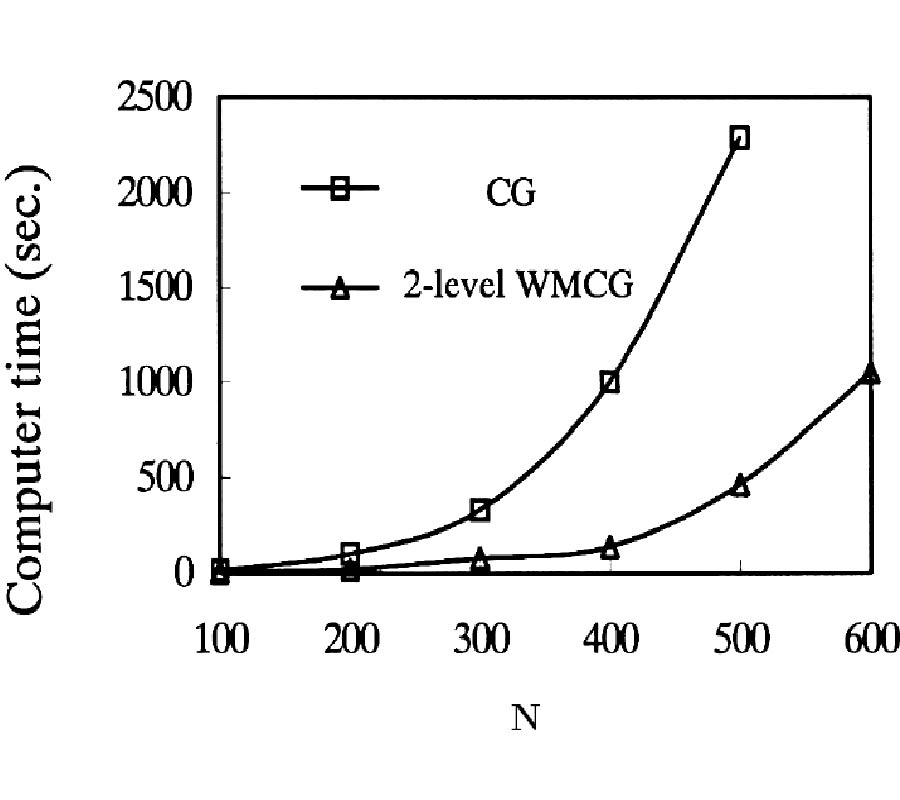Analysis of Electromagnetic Wave Scattering by an Electrically Large Metallic Grating Using Wavelet-Based Algebratic Multigrid Preconditioned Cg Method

R. S. Chen, D. G. Fang, K. F. Tsang, and E. K. N. Yung

An effective wavelet based multigrid preconditioned conjugate gradient method is developed to solve electromagnetic large matrix problem for millimeter wave scattering application. By using wavelet transformation we restrict the large matrix equation to a relative smaller matrix and which can be solved rapidly. The solution is prolonged as the new improvement for the conjugate gradient (CG) method. Numerical result shows that our developed wavelet based multigrid preconditioned CG method can reach large improvement of computational complexity. Due to the automaticity of wavelet transformation, this method is potential to be a block box solver without physical background.

PIER Vol. 31, 69-88, 2001. doi:10.2528/PIER00052903

###Coherence Characteristics of Radar Signals from Rough Soil

X. Luo, J. Askne, G. Smith, and P. Dammert

To understand the mechanisms of decorrelation in interferometric SAR (InSAR) images of bare soil, a model has been developed. Under the Kirchhoff and stationary phase approximations, coherence can be related to the statistical variations of dielectric constant and roughness parameters of surfaces. With the help of an empirical model for the dependence of dielectric constant on soil moisture, coherence due to the inhomogeneity of soil moisture is numerically demonstrated. It has been shown that the decorrelation of the radar signal from rough soil is mainly due to the moisture variability within the resolution cell. The effect of roughness on decorrelation is complex. The effect is negligible compared to that of the dielectric variability for homogeneous resolution cells (no dielectric variability within a resolution cell). However, the coherence depends strongly on the roughness parameters for resolution cells with large moisture variability. It is concluded that the loss of coherence induced by variability of dielectric constant can be related to the relative variation of moisture expressed by the ratio of standard deviation and mean value, and that large relative variations of moisture could lead to much decorrelation. If the moisture variability is small the coherence will be very high even if the values of mean moisture of the two SAR observations are different, which means that coherence can be high in spite of much backscatter differences.

PIER Vol. 31, 55-67, 2001. doi:10.2528/PIER00052202

###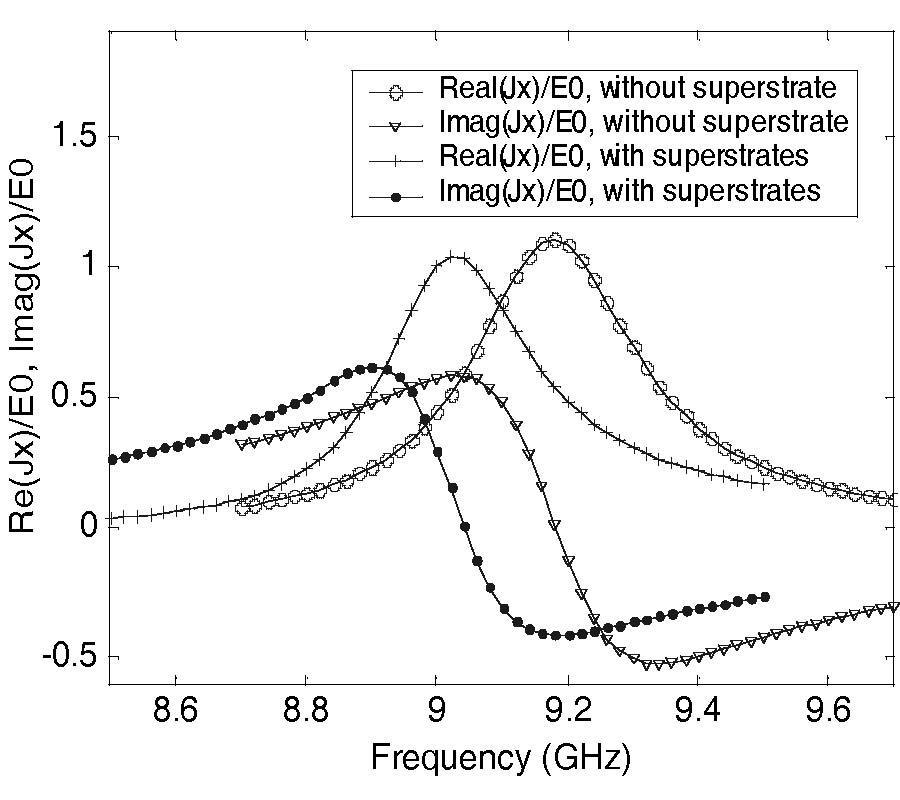Resonant Behaviours of Microstrip Antenna in Multilayered Media: an Efficient Full-Wave Analysis

L. Xia, C.-F. Wang, L.-W. Li, P.-S. Kooi, and M.-S. Leong

This paper presents an efficient analysis of a microstrip antenna in multilayered media using the method of moments (MoM). The general forms of spectral-domain Green’s functions for multilayered media are converted into the spatial-domain Green’s functions using the discrete complex image method (DCIM). With the spatial-domain Green’s functions, the mixed potential integral equation (MPIE) is discretized in spatial domain, and the resultant system of equations is then solved using the biconjugate gradient (BCG) method. As applications, resonant frequencies of microstrip antennas in multilayered media are analysed. The numerical results show that the present method is an efficient and accurate scheme for analysing microstrip antennas in multilayered media.

PIER Vol. 31, 31-54, 2001. doi:10.2528/PIER00041802

###Theoretical Modeling of Multi-Sleeve Monopole Antennas

Z. Shen and R. H. MacPhie

This article presents a numerical technique based on the modal-expansion method for modeling a multi-sleeve monopole antenna fed through an infinite ground-plane by a coaxial transmission line. The modal-expansion analysis is facilitated by introducing a perfectly conducting boundary at a variable height over the ground-plane of the monopole. The resulting structure is then divided into a number of regions and the electromagnetic field components in each region are expanded into the summation of its modal functions. The current distribution over the monopole and sleeve surfaces as well as the antenna's input impedance are computed by finding the expansion coefficients through matching the tangential field components across the regional interfaces. Numerical results for the surface current distribution and input impedance are presented and discussed with emphasis laid on single- and double-sleeve monopole antennas.

PIER Vol. 31, 1-30, 2001. doi:10.2528/PIER00070101

###Finite Difference Time Domain (FDTD) Impedance Boundary Condition for Thin Finite Conducting Sheets

J. J. Akerson, M. A. Tassoudji, Y. E. Yang, and J. A. Kong

A new Impedance Boundary Condition (IBC) for two dimensional Finite Difference Time Domain simulations containing thin, good conductor sheets is presented. The IBC uses a recursive convolution scheme based on approximating the conductor's impedance as a sum of exponentials. The effects of FDTD parameters such as grid size and time step on simulation accuracy are presented. The IBC verification is performed by comparing the quality factors of rectangular resonant structures determined by the FDTD simulation and analytical methods. The IBC is shown to accurately model the conductor loss over a wide frequency range.